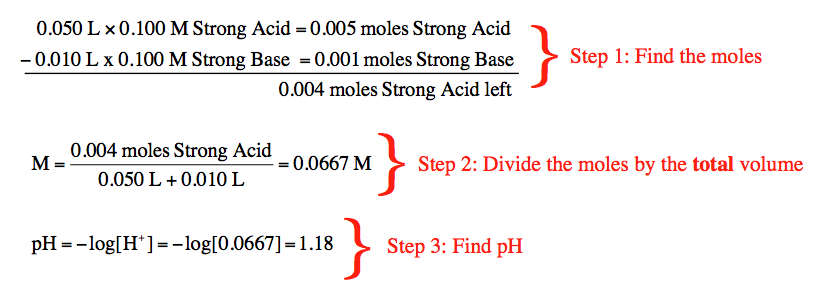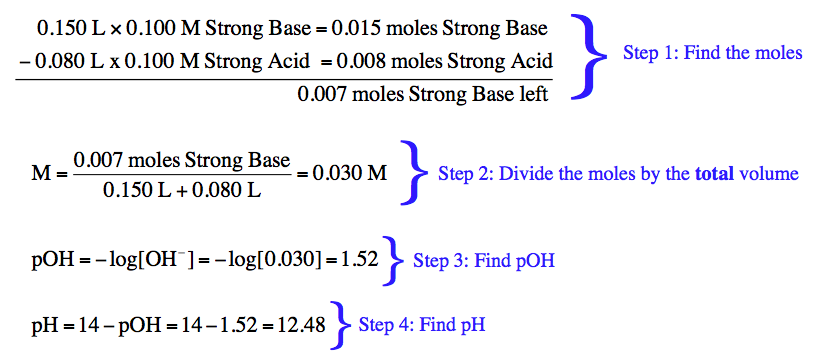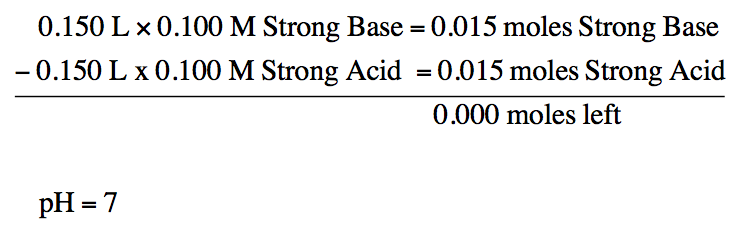Ch.16 - Aqueous Equilibrium WorksheetSee all chapters
 Ch.1 - Intro to General Chemistry 2hrs & 53mins 0% complete Worksheet Ch.2 - Atoms & Elements 2hrs & 49mins 0% complete Worksheet Ch.3 - Chemical Reactions 3hrs & 25mins 0% complete Worksheet BONUS: Lab Techniques and Procedures 1hr & 38mins 0% complete Worksheet BONUS: Mathematical Operations and Functions 47mins 0% complete Worksheet Ch.4 - Chemical Quantities & Aqueous Reactions 3hrs & 30mins 0% complete Worksheet Ch.5 - Gases 3hrs & 47mins 0% complete Worksheet Ch.6 - Thermochemistry 2hrs & 28mins 0% complete Worksheet Ch.7 - Quantum Mechanics 2hrs & 35mins 0% complete Worksheet Ch.8 - Periodic Properties of the Elements 1hr & 57mins 0% complete Worksheet Ch.9 - Bonding & Molecular Structure 2hrs & 5mins 0% complete Worksheet Ch.10 - Molecular Shapes & Valence Bond Theory 1hr & 31mins 0% complete Worksheet Ch.11 - Liquids, Solids & Intermolecular Forces 3hrs & 40mins 0% complete Worksheet Ch.12 - Solutions 2hrs & 17mins 0% complete Worksheet Ch.13 - Chemical Kinetics 2hrs & 22mins 0% complete Worksheet Ch.14 - Chemical Equilibrium 2hrs & 26mins 0% complete Worksheet Ch.15 - Acid and Base Equilibrium 4hrs & 42mins 0% complete Worksheet Ch.16 - Aqueous Equilibrium 3hrs & 48mins 0% complete Worksheet Ch. 17 - Chemical Thermodynamics 1hr & 44mins 0% complete Worksheet Ch.18 - Electrochemistry 2hrs & 58mins 0% complete Worksheet Ch.19 - Nuclear Chemistry 1hr & 33mins 0% complete Worksheet Ch.20 - Organic Chemistry 3hrs 0% complete Worksheet Ch.22 - Chemistry of the Nonmetals 2hrs & 1min 0% complete Worksheet Ch.23 - Transition Metals and Coordination Compounds 1hr & 54mins 0% complete Worksheet

# Strong Acid Strong Base Titrations

See all sections

In a Strong Acid-Strong Base Titration we do not use an ICF Chart or ICE Chart to determine the pH of the solution.

###### Strong Acid-Strong Base Titration

Concept #1: Strong Acid-Strong Base Titration

Example #1: Calculate the pH of the solution resulting from the titration of 75.0 mL of 0.100 M HBrO4 with 55.0 mL of 0.100 M NaNH2

Transcript

Let's take a look here where it says, 'Calculate the pH of the solution resulting from the titration of 75.0 mL of 0.100 M HBrO4 with 55 mL of 0.100 M NaNH2. Remember, this right here is a strong acid. This right here is a strong base. What we're going to say here is because both are strong, we don't have to use an ICE, we don’t have to use an ICF.
What do we do instead? We're going to say step one is find the moles. Remember, how do we find moles? Remember, moles equals molarity times liters. Divide the mL by a thousand, multiply them times their lit on their molarities.
Now, remember for the base, for the base you're always supposed to check how many OH minuses, NH2 minuses, H minuses and O2 minuses you have to get the correct concentration. Here we only have one NH2, so we don't have to worry about multiplying the concentration by anything, we can keep it as it is.
When we do this, we're going to say 0.075 L times 0.100 M HBrO4 equals 0.0075 M of HBrO4. Then we do the same thing with the other one, so we have that. Now that we found their moles, we're going to subtract the large one by the smaller one. What you're going to say is now you have this much left of your strong acid. That's all you're going to say, that's how much you have left of your strong acid.
Now step two, you're going to find molarity of whatever you have left. How do we find molarity? Well, we're going to take the moles that we just found and divide them by the total volume. Those two liters added up together will give you my total volume.
We're going to say 0.0020 moles of HBrO4 divide by the total volume we use, 0.0075 L plus 0.055 L. When we divide those together, that's going to give me, 0.0154 M HBrO4. Remember, since this is a strong acid, to find pH we don't need to do an ICE Chart. Since this is a strong acid, just take the negative log. Negative log of a strong acid will give me my pH.
So we plug in the concentration here and at the end, we scroll up some, at the end, what is that going to give me, my pH will be 1.81. So it's as simple as that. If both are strong, this is a great situation, first find the moles of both.
Remember look at the correct molarity of the strong base. If you have to multiply it by two because there's two OH or there's two NH2 or there's two Hs, H minuses, then you do that before you change everybody into moles.
Once you find moles, you subtract the larger moles from the smaller moles, whoever is left behind, whether it be a strong acid or a strong base, you immediately change it into molarity. If you have the molarity of a strong acid, take the negative log to find pH.
If you have the molarity of a base, so strong base then take the negative log of that, but that will give us pOH. So remember strong base if you take the negative log, it gives us pOH. If you have pOH, you're going to take that answer that you find and subtract it from 14 and that will give you pH. It's as simple as that.
Just remember the steps. Step one—find moles, step two—find molarity, step three—I guess you just take the negative log or whatever your answer is to find neither, pH or pOH. That's all you really have to do, there's no need of any type of chart.
Now that we've done this one, I want you guys to attempt to do the next one on your own as practice. So just take the same method that we used above in order to find it. Make sure that you get the correct concentrations of everyone before change everyone into moles. As long as you can remember that, you'll be able to solve this question. Good luck, guys.

If after the titration there is an excess of strong acid left then we can use its concentration to find the pH.If after the titration there is an excess of strong base then we can use its concentration to find pOH initially.Practice: Calculate the pH of the solution resulting from the mixing of 175.0 mL of 0.250 M HNO3 with 75.0 mL of 0.200M Ba(OH)2.

If there is an equal amount of moles for both the strong acid and strong base then the pH of the solution will be 7.